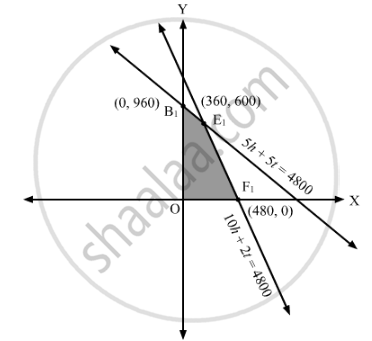# A Publisher Sells a Hard Cover Edition of a Text Book for Rs 72.00 and Paperback Edition of the Same Ext for Rs 40.00. - Mathematics

Sum

A publisher sells a hard cover edition of a text book for Rs 72.00 and paperback edition of the same ext for Rs 40.00. Costs to the publisher are Rs 56.00 and Rs 28.00 per book respectively in addition to weekly costs of Rs 9600.00. Both types require 5 minutes of printing time, although hardcover requires 10 minutes binding time and the paperback requires only 2 minutes. Both the printing and binding operations have 4,800 minutes available each week. How many of each type of book should be produced in order to maximize profit?

#### Solution

Let the sale of hand cover edition be 'h' and that of paperback editions be 't'.

SP of a hard cover edition of the textbook = Rs 72
SP of a paperback edition of the textbook = Rs 40

Cost to the publisher for hard cover edition = Rs 56
Cost to the publisher for a paperback edition = Rs 28
Weekly cost to the publisher = Rs 9600
Profit to be maximised, Z = (72 − 56) h + (40 − 28) t − 9600
⇒ Z = 16h + 12t − 9600
5 (h + t) ≤ 4800
10 h + 2t ≤ 4800The corner points are O(0, 0), B1(0, 960),  E1(360, 600) and F1(480, 0).
The values of Z at these corner points are as follows:

 Corner point Z = 16h + 12t − 9600 O −9600 B1 1920 E1 3360 F1 −1920

The maximum value of Z is 3360 which is attained at E1(360, 600).
The maximum profit is 3360 which is obtained by selling 360 copies of hardcover edition and 600 copies of paperback edition.

Concept: Graphical Method of Solving Linear Programming Problems
Is there an error in this question or solution?

#### APPEARS IN

RD Sharma Class 12 Maths
Chapter 30 Linear programming
Exercise 30.4 | Q 15 | Page 52